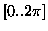Next: Results Up: The Problem Spaces Previous: Finding Trigonometric Identities

## Symbolic Integration

Thirdly, we have applied our system to a symbolic integration problem as summarised in Table 3. Symbolic Integration involves finding a function which is the integral of the given curve, in this case Cos (X) + 2X + 1. The system is given a set of input and output pairs, and must determine the function that maps one onto the other. The particular function examined was Cos (X) + 2X + 1 with the input values in the rangeand, the target integral curve is Sin(X)+X+X2. Similarly to the Symbolic Regression problem described earlier, this problem used the same BNF grammar, including the terminal operators +, *, /,-, Sin, Cos, Exp and Log. The addition, multiplication and Sin operators would have been suffcient to solve this problem but, again to avoid biasing the system other terminal operators were included.

Table 3: Grammatical Evolution Tableau for Symbolic Integration
 Objective : Find a function, in symbolic form, that is the integral of a curve presented either as a mathematical expression or as a given finite sample of points (xi,yi) Terminal Operands: X (the independent variable) Terminal Operators The binary operators +, *, /,- and the unary operators Sin, Cos, Exp and Log Fitness cases The given sample of 50 data points in the interval [] Raw Fitness The sum, taken over the 50 fitness cases, of the absolute value of the difference between the individual genetically produced function fj(xi) at the domain point xi and the value of the numerical integral I(xi) Standardised Fitness Same as raw fitness Hits The number of fitness cases for which the error is less than 0.01 Wrapper Standard productions to generate C functions Parameters M = 500, G = 51Next: Results Up: The Problem Spaces Previous: Finding Trigonometric Identities
root
1998-10-02